Ex 15.1

Chapter 15 Class 9 Probability (Term 2)
Serial order wise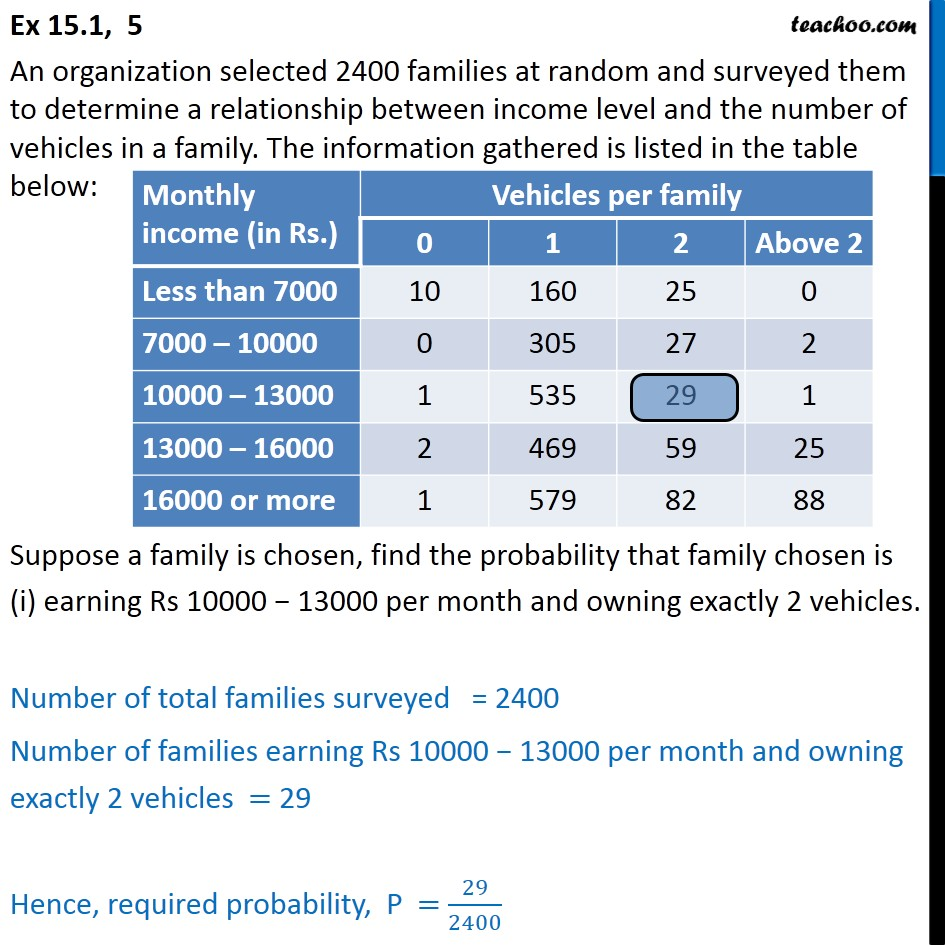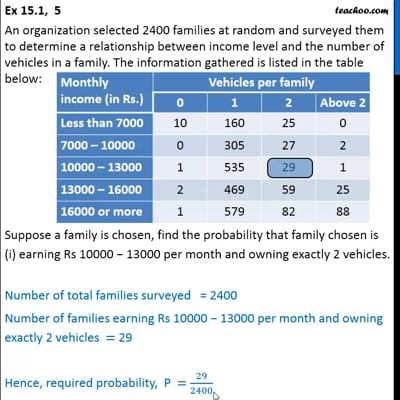This video is only available for Teachoo black users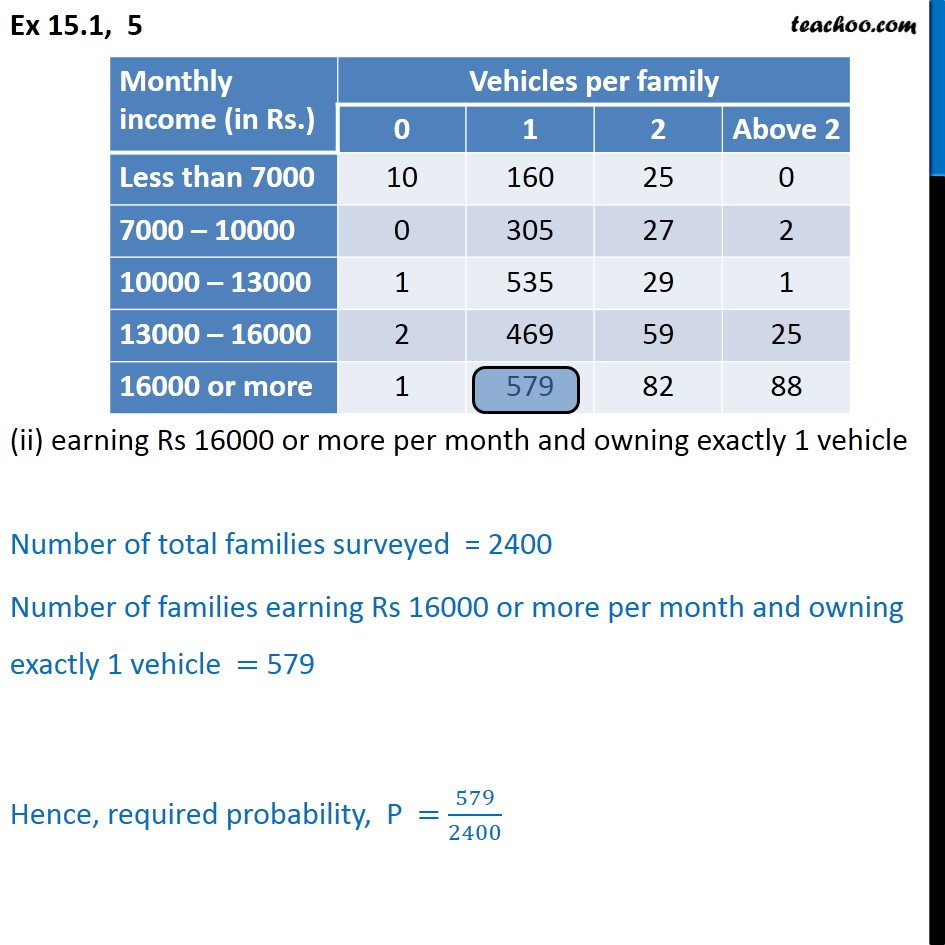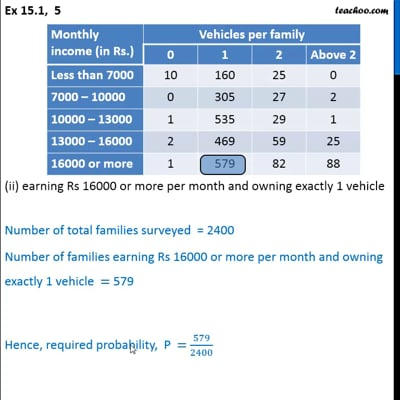This video is only available for Teachoo black users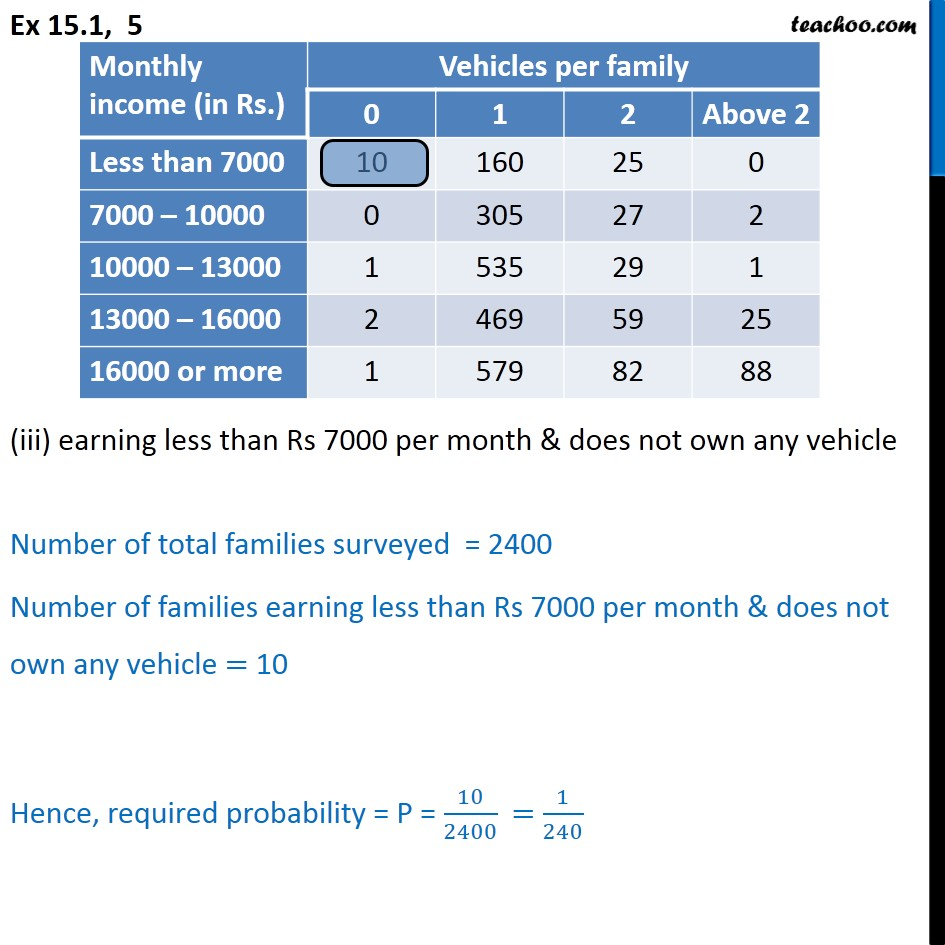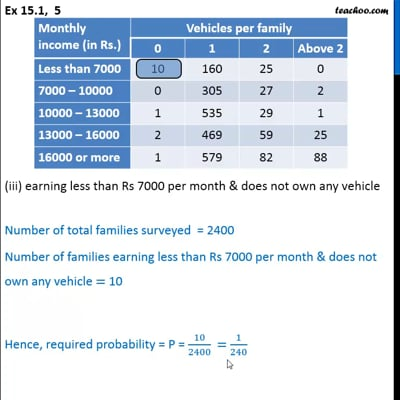This video is only available for Teachoo black users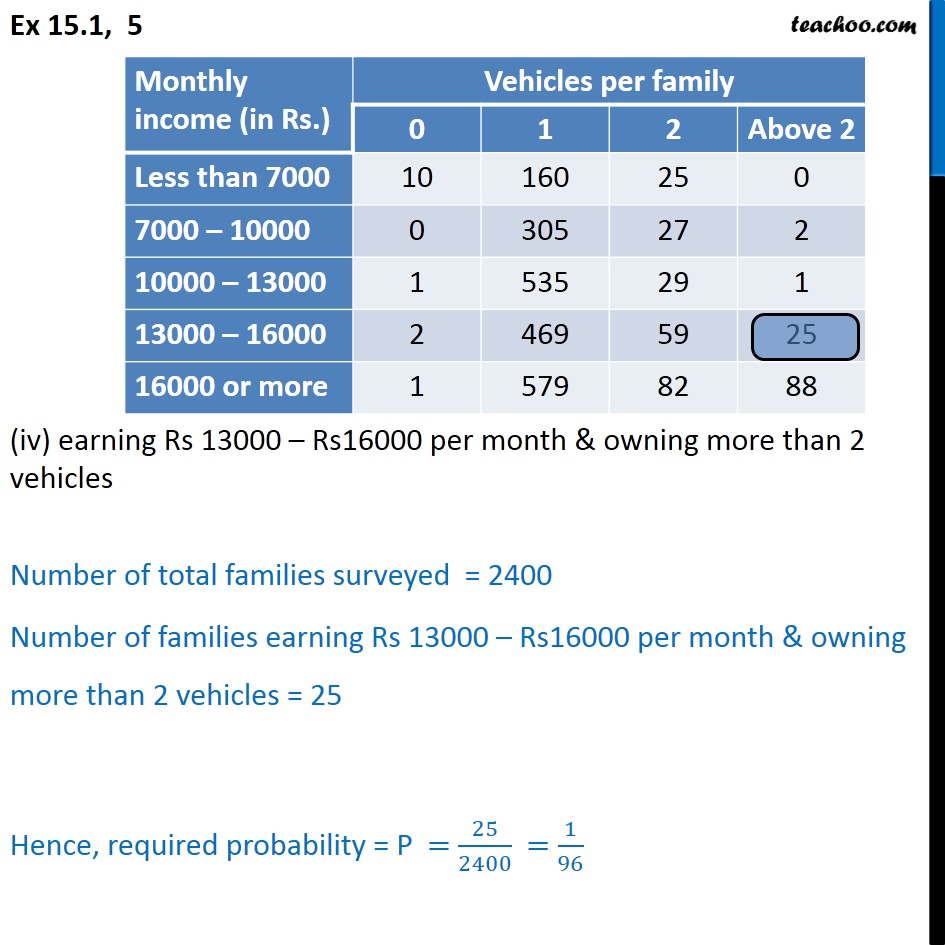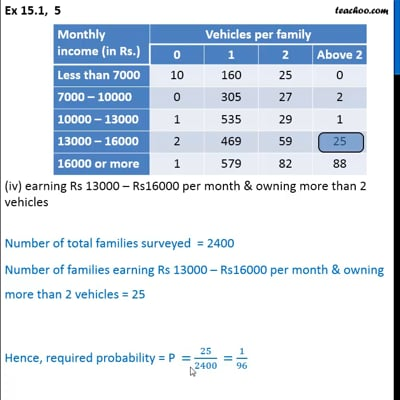This video is only available for Teachoo black users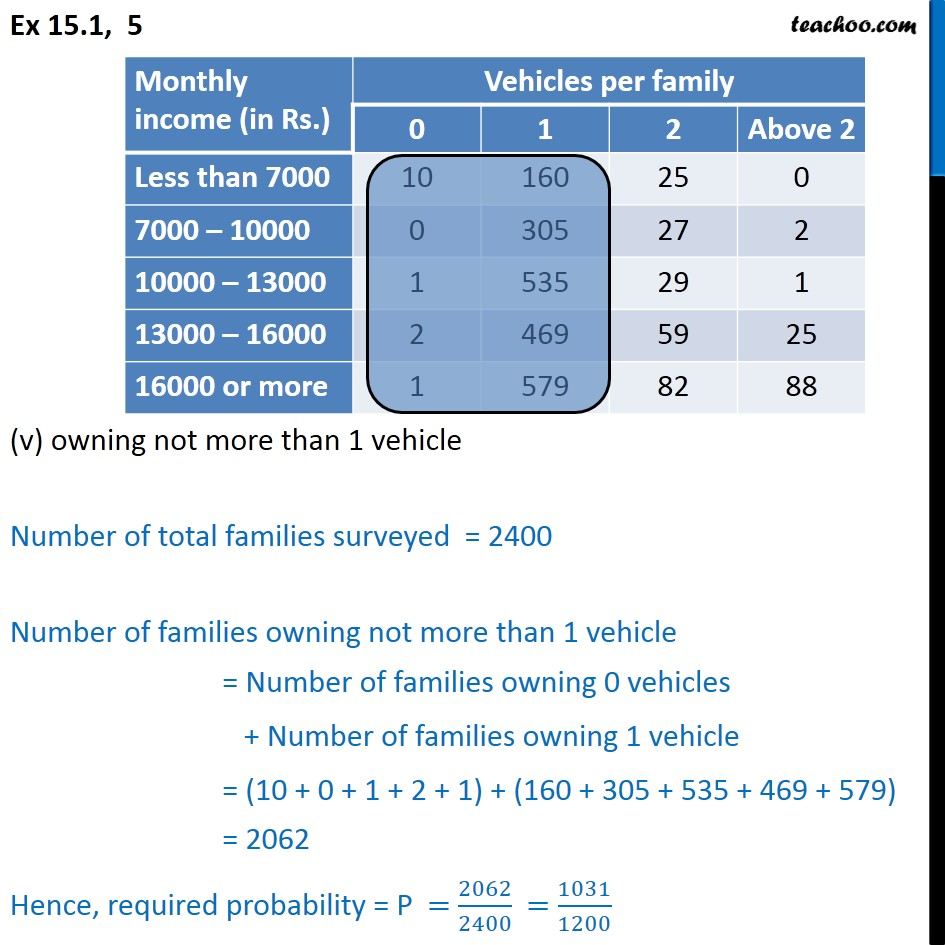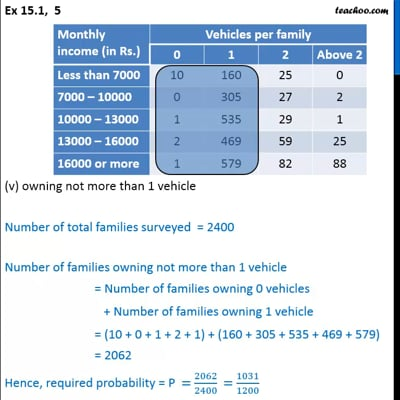This video is only available for Teachoo black users

### Transcript

Ex 15.1, 5 An organization selected 2400 families at random and surveyed them to determine a relationship between income level and the number of vehicles in a family. The information gathered is listed in the table below: Suppose a family is chosen, find the probability that family chosen is (i) earning Rs 10000 − 13000 per month and owning exactly 2 vehicles. Number of total families surveyed = 2400 Number of families earning Rs 10000 ­− 13000 per month and owning exactly 2 vehicles = 29 Hence, required probability, P = 29/2400 Ex 15.1, 5 (ii) earning Rs 16000 or more per month and owning exactly 1 vehicle Number of total families surveyed = 2400 Number of families earning Rs 16000 or more per month and owning exactly 1 vehicle = 579 Hence, required probability, P = 579/2400 Ex 15.1, 5 (iii) earning less than Rs 7000 per month & does not own any vehicle Number of total families surveyed = 2400 Number of families earning less than Rs 7000 per month & does not own any vehicle = 10 Hence, required probability = P = 10/2400 = 1/240 Ex 15.1, 5 (iv) earning Rs 13000 – Rs16000 per month & owning more than 2 vehicles Number of total families surveyed = 2400 Number of families earning Rs 13000 – Rs16000 per month & owning more than 2 vehicles = 25 Hence, required probability = P = 25/2400 = 1/96 Ex 15.1, 5 (v) owning not more than 1 vehicle Number of total families surveyed = 2400 Number of families owning not more than 1 vehicle = Number of families owning 0 vehicles + Number of families owning 1 vehicle = (10 + 0 + 1 + 2 + 1) + (160 + 305 + 535 + 469 + 579) = 2062 Hence, required probability = P = 2062/2400 = 1031/1200